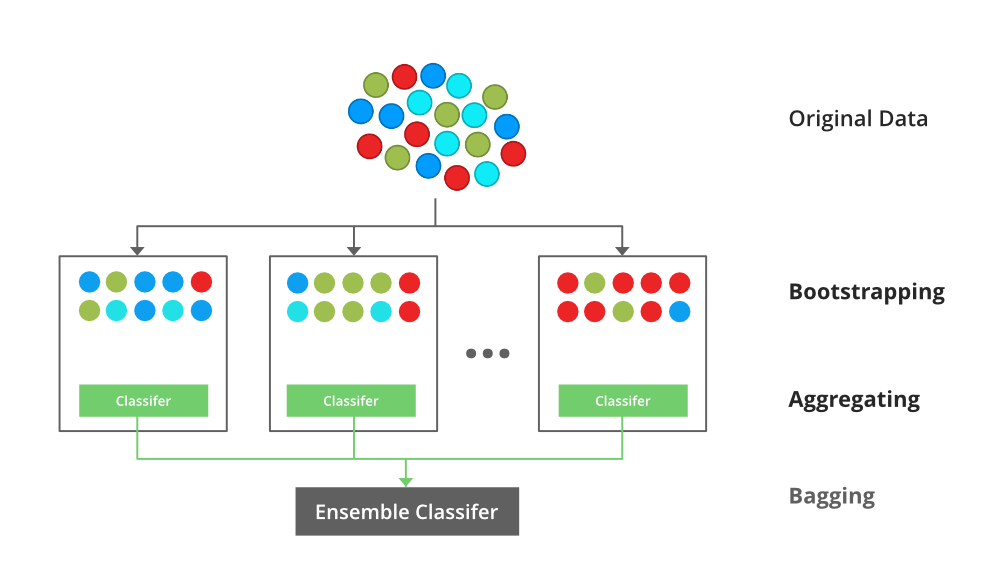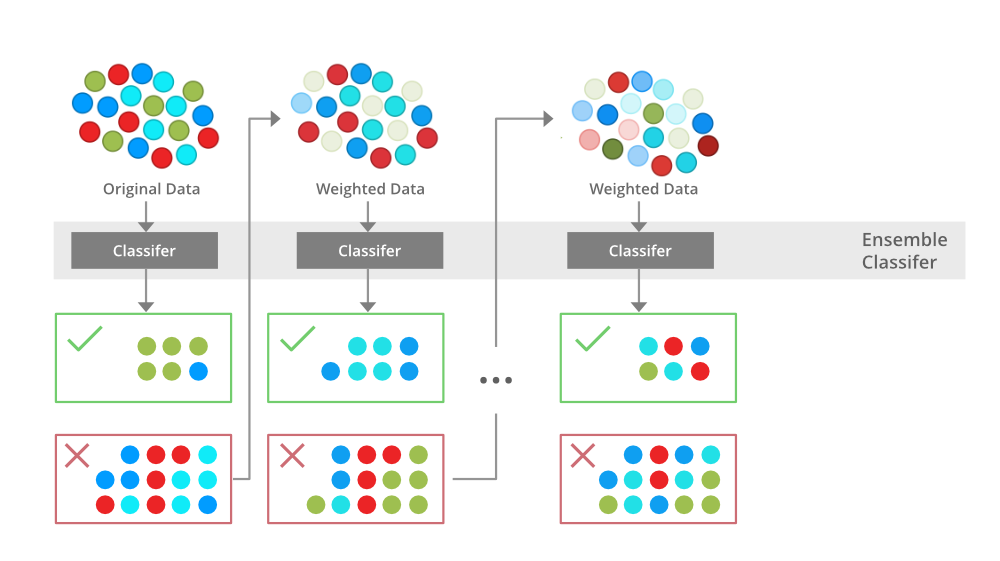# Bagging vs Boosting in Machine Learning

• Difficulty Level : Medium
• Last Updated : 01 Jun, 2022

As we know, Ensemble learning helps improve machine learning results by combining several models. This approach allows the production of better predictive performance compared to a single model. Basic idea is to learn a set of classifiers (experts) and to allow them to vote. Bagging and Boosting are two types of Ensemble Learning. These two decrease the variance of a single estimate as they combine several estimates from different models. So the result may be a model with higher stability. Let’s understand these two terms in a glimpse.

1. Bagging: It is a homogeneous weak learners’ model that learns from each other independently in parallel and combines them for determining the model average.
2. Boosting: It is also a homogeneous weak learners’ model but works differently from Bagging. In this model, learners learn sequentially and adaptively to improve model predictions of a learning algorithm.

Let’s look at both of them in detail and understand the Difference between Bagging and Boosting.

## Bagging

Bootstrap Aggregating, also known as bagging, is a machine learning ensemble meta-algorithm designed to improve the stability and accuracy of machine learning algorithms used in statistical classification and regression. It decreases the variance and helps to avoid overfitting. It is usually applied to decision tree methods. Bagging is a special case of the model averaging approach.

Description of the Technique

Suppose a set D of d tuples, at each iteration i, a training set Di of d tuples is selected via row sampling with a replacement method (i.e., there can be repetitive elements from different d tuples) from D (i.e., bootstrap). Then a classifier model Mi is learned for each training set D < i. Each classifier Mi returns its class prediction. The bagged classifier M* counts the votes and assigns the class with the most votes to X (unknown sample).

Implementation Steps of Bagging

• Step 1: Multiple subsets are created from the original data set with equal tuples, selecting observations with replacement.
• Step 2: A base model is created on each of these subsets.
• Step 3: Each model is learned in parallel with each training set and independent of each other.
• Step 4: The final predictions are determined by combining the predictions from all the models.An illustration for the concept of bootstrap aggregating (Bagging)

Example of Bagging

The Random Forest model uses Bagging, where decision tree models with higher variance are present. It makes random feature selection to grow trees. Several random trees make a Random Forest.

## Boosting

Boosting is an ensemble modeling technique that attempts to build a strong classifier from the number of weak classifiers. It is done by building a model by using weak models in series. Firstly, a model is built from the training data. Then the second model is built which tries to correct the errors present in the first model. This procedure is continued and models are added until either the complete training data set is predicted correctly or the maximum number of models is added.

Boosting Algorithms

There are several boosting algorithms. The original ones, proposed by Robert Schapire and Yoav Freund were not adaptive and could not take full advantage of the weak learners. Schapire and Freund then developed AdaBoost, an adaptive boosting algorithm that won the prestigious Gödel Prize. AdaBoost was the first really successful boosting algorithm developed for the purpose of binary classification. AdaBoost is short for Adaptive Boosting and is a very popular boosting technique that combines multiple “weak classifiers” into a single “strong classifier”.

Algorithm:

1. Initialise the dataset and assign equal weight to each of the data point.
2. Provide this as input to the model and identify the wrongly classified data points.
3. Increase the weight of the wrongly classified data points and decrease the weights of correctly classified data points. And then normalize the weights of all data points.
4. if (got required results)
Goto step 5
else
Goto step 2
5. EndAn illustration presenting the intuition behind the boosting algorithm, consisting of the parallel learners and weighted dataset.

### Similarities Between Bagging and Boosting

Bagging and Boosting, both being the commonly used methods, have a universal similarity of being classified as ensemble methods. Here we will explain the similarities between them.

1. Both are ensemble methods to get N learners from 1 learner.
2. Both generate several training data sets by random sampling.
3. Both make the final decision by averaging the N learners (or taking the majority of them i.e Majority Voting).
4. Both are good at reducing variance and provide higher stability.

### Differences Between Bagging and Boosting

My Personal Notes arrow_drop_up